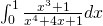## An integral that Maxima (and you) can do better than Mathematica

January 11th, 2008 | Categories: general math, mathematica | Tags:

How good is your symbolic integral calculus? Do you think that you can do better than Mathematica? Let’s see – try and evaluate the following (there is a hint in the comments if you get stuck).My integration skills are a little rusty but I found the solution, log(6)/4, without too much difficulty (I have picked up the habit of writing log(x) when I mean ln(x) from using computer algebra packages too much) . Let’s see how Mathematica handles the same integration. Plugging the following command into version 6

Integrate[(x^3 + 1)/(x^4 + 4*x + 1), {x, 0, 1}]

gives a solution of

(RootSum[1 + 4*#1 + #1^4 & , Log[1 – #1]/(1 + #1^3) & ] -RootSum[1 + 4*#1 + #1^4 & , Log[-#1]/(1 + #1^3) & ] +
RootSum[1 + 4*#1 + #1^4 & ,(Log[1 – #1]*#1^3)/(1 + #1^3) & ] -RootSum[1 + 4*#1 + #1^4 & , (Log[-#1]*#1^3)/(1 + #1^3) & ])/4

Ugh! Applying the FullSimplify command doesn’t help so it seems that this is the best that Mathematica can do at the moment. If you evaluate this expression numerically then it agrees with the symbolic result but I think you would agree that Mathematica has not done a very good job here.

I found this integral while looking through the changelog of the latest version of Maxima – an open source mathematics package. If you try and evaluate it in pre-5.14 versions of Maxima then it will appear to hang (actually it will return a result eventually if you leave it long enough and have enough memory but it makes the Mathematica result look positively pretty). This has been fixed in version 5.14 and now issuing the command

integrate ((x^3 + 1)/(x^4 + 4*x + 1),x,0,1);

gives the result you would expect. So what went wrong – why does such a simple integral cause problems with these powerful software packages? The answer can be found in the full Maxima bug report for this issue – I will let you read it yourself if you are interested but in a nutshell pre 5.14 versions of Maxima were attempting to use a technique from complex analysis called contour integration to evaluate this integral. Contour integration is an amazingly useful technique that can be used to evaluate all sorts of definite integrals that are very difficult to do via other methods but using it in this case was a bad idea. It is possible that Mathematica tried to evaluate the integral in the same way but since it is closed source only the developers at Wolfram know the answer to that.

So this has been fixed in Maxima and I imagine that it will only be a matter of time before it is fixed in Mathematica but until that happens why not give this integral to your students and show them that, sometimes at least, they can do calculus better than Mathematica?

1. The hint for doing the integral by hand is to make the substitution

u = x^4 + 4x + 1

2. For what it’s worth, Maple 10 takes

int((x^3+1)/(x^4+4*x+1), x=0..1);

and outputs

1/4*ln(2)+1/4*ln(3).

3. Thanks for that Isabel – Matlab returns the same when you use its symbolic toolbox since that is based on the Maple front end.

4. Well, Mathematica isn’t using contour integration here. The form of the answer suggests that it’s a Lazard/Rioboo/Rothstein/Trager-like algorithm (the differences in this family are unimportant; they all give similar-looking answers). And, most importantly, they ALWAYS work on rational polynomials, even if you can’t factor the denominator.

What surprises me the most here is that quartics are always soluble, so why didn’t Mathematica go to the trouble of doing that, then simplifying?

5. Try this:

Integrate[(x^3 + 1)/(x^4 + 4*x + 1), {x, 0, 1}]//Normal//RootReduce//FullSimplify

I’m not sure why Mathematica prefers to use the RootSum object here, but getting rid of it proves not to be too arduous.

6. Hi Ben

Thanks for that but it’s a shame that you need to post-process the result of Integrate with 3 different commands in order to get such an elementary result.

Cheers,
Mike

7. Well, it’s a shame only in the sense that it’s unfortunate that CAS doesn’t have the mathematical intuition of a human. The algorithms used in such software are preferably the most general possible, and tend to be those most efficiently applied by a concrete series of lexical transformations. Hence, it’s quite normal to see combinations of Root and RootSum objects where, when doing a problem by hand, one might have used a simpler form in terms of transcendental functions.

I would argue that the requirement for a Mathematica user to know that Normal, RootReduce, and FullSimplify are standard tools for working with such objects is very much equivalent to the requirement that a pencil-and-paper mathematician realises that substitution is the most straightforward approach. That these “tricks” differ between the two situations is really just a reflection of the different solutiuon strategies in each case–this may well confound the novice CAS user or the novice mathematician, but it would be expected that one becomes equally comfortable and confident with either approach given sufficient experience of both.

In a more concrete sense, standard mathematical texts give advice for and examples of integration by substitution, while the Mathematica documentation advises and exemplifies transformation of Root objects into more recognisable forms. In my view, both are equally valid and equally accessible references if one requires help in solving problems such as this. Regardless of which source one chooses, the problem is easily solved in two or three lines of working.

8. Ben – A good point well made.
Thanks,
Mike

9. if u make:
f[x_] = Integrate[(x^3 + 1)/(x^4 + 4 x + 1), x]

f – f

you will have what u want.

10. Also,